2017-10-16 17:27:31 divergent63 阅读数 292
• ###### 玩转深度学习实战教程

玩转深度学习视频培训课程，详细讲解深度学习的原理和利用深度学习框架TensorFlow进行项目实战。课程通过Kaggle竞赛平台的Titanic问题讲解TensorFlow的基本用法以及问题处理的常用技巧，讲解深度学习图像领域的卷积神经网络CNN和多个经典的网络架构、CNN的应用，讲解自然语言处理领域的RNN、LSTM以及它们的多种变种结构，构建语言模型和对话机器人，介绍损失函数和优化深度学习算法在TensorFlow中的实现。

3209 人正在学习 去看看 王而川

win10-anaconda3-py3.5-tensorflow1.0.1-keras2.0.5

import tensorflow as tf
import numpy as np
import matplotlib.pyplot as plt

def add_layer(inputs, in_size, out_size, n_layer, activation_function=None):
layer_name = ‘layer%s’ % n_layer
with tf.name_scope(‘layer’):
with tf.name_scope(‘weights’):
Weights = tf.Variable(tf.random_normal([in_size, out_size]), name=’W’)
tf.summary.histogram(layer_name + ‘/weights’, Weights)
with tf.name_scope(‘biases’):
biases = tf.Variable(tf.zeros([1, out_size]) + 0.1, name=’b’)
tf.summary.histogram(layer_name + ‘/biases’, biases)
with tf.name_scope(‘Wx_plus_b’):
Wx_plus_b = tf.add(tf.matmul(inputs, Weights), biases, name=’Wpb’)
if activation_function is None:
outputs = Wx_plus_b

``````    else:
outputs = activation_function(Wx_plus_b)
tf.summary.histogram(layer_name + '/outputs', outputs)
return outputs
``````

with tf.name_scope(‘inputs’):
xs = tf.placeholder(tf.float32, [None, 1], name=’x_input’)
ys = tf.placeholder(tf.float32, [None, 1], name=’y_input’)

x_data = np.linspace(-1.0, 1.0, 300, dtype=np.float32)[:, np.newaxis]
noise = np.random.normal(0.0, 0.05, x_data.shape)
y_data = np.square(x_data) + 0.5 + noise

l1 = add_layer(xs, 1, 10, n_layer=1, activation_function=tf.nn.relu)
prediction = add_layer(l1, 10, 1, n_layer=2, activation_function=None)

with tf.name_scope(‘loss’):
loss = tf.reduce_mean(tf.reduce_sum(tf.square(ys - prediction), reduction_indices=), name=’L’)
tf.summary.scalar(‘loss’, loss)

with tf.name_scope(‘train’):

# init = tf.initialize_all_variables()

init = tf.global_variables_initializer()
with tf.Session() as sess:
sess.run(init)
fig = plt.figure()
ax.scatter(x_data, y_data)
plt.show(block=False)
merged = tf.summary.merge_all()
writer = tf.summary.FileWriter(‘I:/CASIA/deeplearning/tensorBoard/tmp/TB_Graph/1’, sess.graph)
”’tensorboard –logdir=I:/CASIA/deeplearning/tensorBoard/tmp/TB_Graph/1”’
for i in range(10):
sess.run(train_step, feed_dict={xs:x_data, ys : y_data})
if i % 50 == 0:
try:
ax.lines.remove(lines)
except Exception:
pass
result, prediction_value = sess.run([merged, prediction], feed_dict={xs:x_data, ys:y_data})
lines = ax.plot(x_data, prediction_value, ‘r-‘, lw=5)
plt.pause(0.1)

D:\software\Anconda_python\A3\python.exe I:/CASIA/deeplearning/LSTM/selfAlgorithm/TB_Graph.py
E c:\tf_jenkins\home\workspace\release-win\device\cpu\os\windows\tensorflow\core\framework\op_kernel.cc:943] OpKernel (‘op: “BestSplits” device_type: “CPU”’) for unknown op: BestSplits
E c:\tf_jenkins\home\workspace\release-win\device\cpu\os\windows\tensorflow\core\framework\op_kernel.cc:943] OpKernel (‘op: “CountExtremelyRandomStats” device_type: “CPU”’) for unknown op: CountExtremelyRandomStats
E c:\tf_jenkins\home\workspace\release-win\device\cpu\os\windows\tensorflow\core\framework\op_kernel.cc:943] OpKernel (‘op: “FinishedNodes” device_type: “CPU”’) for unknown op: FinishedNodes
E c:\tf_jenkins\home\workspace\release-win\device\cpu\os\windows\tensorflow\core\framework\op_kernel.cc:943] OpKernel (‘op: “GrowTree” device_type: “CPU”’) for unknown op: GrowTree
E c:\tf_jenkins\home\workspace\release-win\device\cpu\os\windows\tensorflow\core\framework\op_kernel.cc:943] OpKernel (‘op: “ReinterpretStringToFloat” device_type: “CPU”’) for unknown op: ReinterpretStringToFloat
E c:\tf_jenkins\home\workspace\release-win\device\cpu\os\windows\tensorflow\core\framework\op_kernel.cc:943] OpKernel (‘op: “SampleInputs” device_type: “CPU”’) for unknown op: SampleInputs
E c:\tf_jenkins\home\workspace\release-win\device\cpu\os\windows\tensorflow\core\framework\op_kernel.cc:943] OpKernel (‘op: “TopNInsert” device_type: “CPU”’) for unknown op: TopNInsert
E c:\tf_jenkins\home\workspace\release-win\device\cpu\os\windows\tensorflow\core\framework\op_kernel.cc:943] OpKernel (‘op: “TopNRemove” device_type: “CPU”’) for unknown op: TopNRemove
E c:\tf_jenkins\home\workspace\release-win\device\cpu\os\windows\tensorflow\core\framework\op_kernel.cc:943] OpKernel (‘op: “TreePredictions” device_type: “CPU”’) for unknown op: TreePredictions
E c:\tf_jenkins\home\workspace\release-win\device\cpu\os\windows\tensorflow\core\framework\op_kernel.cc:943] OpKernel (‘op: “UpdateFertileSlots” device_type: “CPU”’) for unknown op: UpdateFertileSlots
D:\software\Anconda_python\A3\lib\site-packages\matplotlib\backend_bases.py:2453: MatplotlibDeprecationWarning: Using default event loop until function specific to this GUI is implemented
warnings.warn(str, mplDeprecation)

Process finished with exit code 0

2018-01-03 21:00:50 Tong_T 阅读数 54002
• ###### 玩转深度学习实战教程

玩转深度学习视频培训课程，详细讲解深度学习的原理和利用深度学习框架TensorFlow进行项目实战。课程通过Kaggle竞赛平台的Titanic问题讲解TensorFlow的基本用法以及问题处理的常用技巧，讲解深度学习图像领域的卷积神经网络CNN和多个经典的网络架构、CNN的应用，讲解自然语言处理领域的RNN、LSTM以及它们的多种变种结构，构建语言模型和对话机器人，介绍损失函数和优化深度学习算法在TensorFlow中的实现。

3209 人正在学习 去看看 王而川

• 深度学习是基于机器学习延伸出来的一个新的领域，由以人大脑结构为启发的神经网络算法为起源加之模型结构深度的增加发展，并伴随大数据和计算能力的提高而产生的一系列新的算法。• 其概念由著名科学家Geoffrey Hinton等人在2006年和2007年在《Sciences》等上发表的文章被提出和兴起。• 深度学习，作为机器学习中延伸出来的一个领域，被应用在图像处理与计算机视觉，自然语言处理以及语音识别等领域。自2006年至今，学术界和工业界合作在深度学习方面的研究与应用在以上领域取得了突破性的进展。以ImageNet为数据库的经典图像中的物体识别竞赛为例，击败了所有传统算法，取得了前所未有的精确度。2. 莫烦 Python（https://morvanzhou.github.io/）：一个志同道合的机器学习爱好者。

2018-03-25 23:01:25 do_best_ 阅读数 6454
• ###### 玩转深度学习实战教程

玩转深度学习视频培训课程，详细讲解深度学习的原理和利用深度学习框架TensorFlow进行项目实战。课程通过Kaggle竞赛平台的Titanic问题讲解TensorFlow的基本用法以及问题处理的常用技巧，讲解深度学习图像领域的卷积神经网络CNN和多个经典的网络架构、CNN的应用，讲解自然语言处理领域的RNN、LSTM以及它们的多种变种结构，构建语言模型和对话机器人，介绍损失函数和优化深度学习算法在TensorFlow中的实现。

3209 人正在学习 去看看 王而川

## 五、实验心得与体会

1、了解深度学习的基本原理，能够解释深度学习原理；

2、能够使用深度学习开源工具tensorflow识别图像中的数字，对实验性能进行分析；

3、了解图像识别的基本原理。

【深度学习】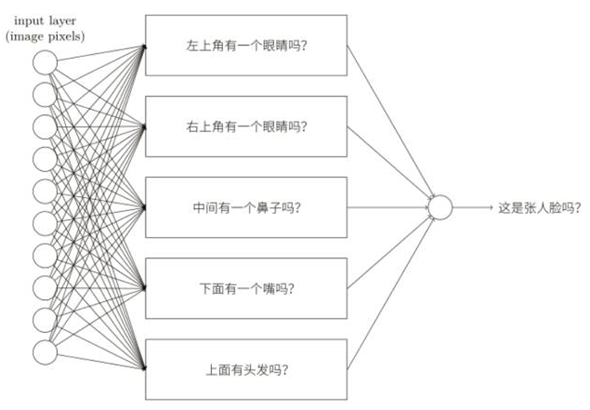【将MNIST二进制数据集转化为图片形式】

``````// 导入input_data这个类
from tensorflow.examples.tutorials.mnist import input_data
``````

（1）55,000个训练样本（用来训练模型）
（2）10,000个测试样本（用来测试模型，避免过拟合）
（3）5,000个验证样本（用来验证超参数）
(一般在机器学习的建模中，都需要准备这3类数据集。)

（1）手写数字图片，记做x
（2）标签，记做y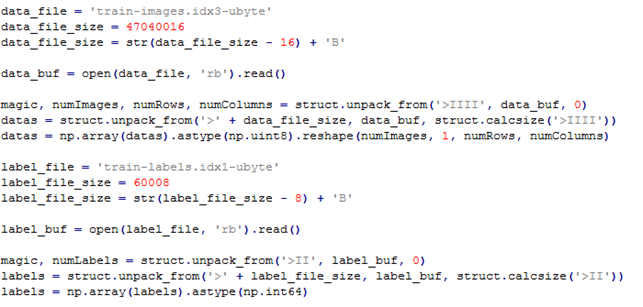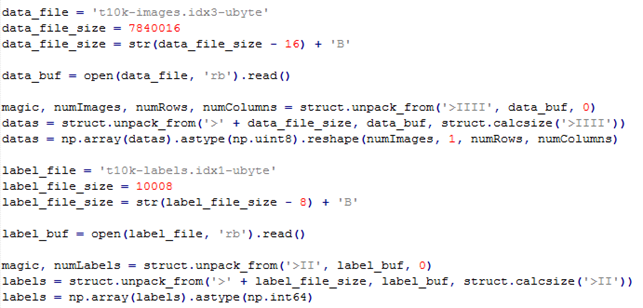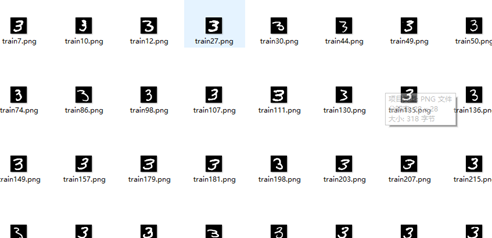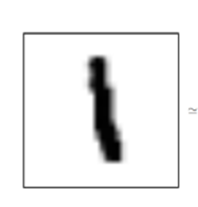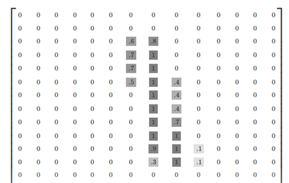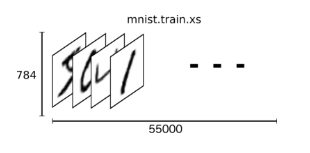【建立多分类模型】

Softmax Regressions原理

Softmax Regressions主要有2步：

1、分别将输入数据属于某个类别的证据相加；

2、将这个证据转换成概率。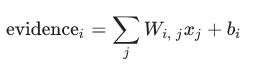i表示第i类，j表示输入的这张图片的第j个像素，也就是求将每个像素乘以它的权重w，在加上偏执项的和。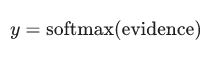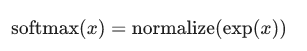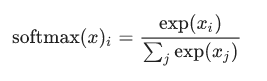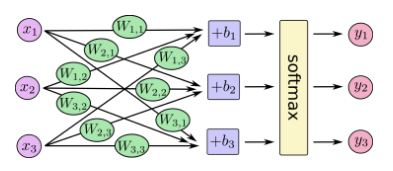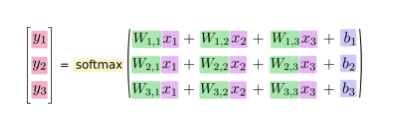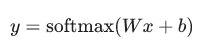【深度神经网络实现图像识别】

1）使用tensorflow，实现导入tensorflow的库

Import tensorflowas tf

2）为输入数据x创建占位符

x =tf.placeholder(tf.float32, [None, 784])

3）创建两个权重变量

W和b是在训练过程中不断改变不断优化的，使用variable来创建：

W =tf.Variable(tf.zeros([784, 10]))

b =tf.Variable(tf.zeros())

4）建立损失函数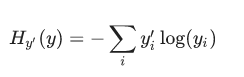y表示模型预测出来的概率分布，y’表示真实的类别概率分布（就是之间one-hot编码之后的标签）。yi表示预测为第i个类的概率， yi’表示真实属于i类的概率（只有i类为1，其余为0）

``````// 为真实的标签添加占位符
y_ = tf.placeholder(tf.float32, [None, 10])

// 创建交叉熵函数
cross_entropy = tf.reduce_mean(-tf.reduce_sum(y_ * tf.log(y), reduction_indices=))
``````

5）使用BP算法优化参数

6）建立softmax模型

y =tf.nn.softmax(tf.matmul(x, W) + b)

7）运行迭代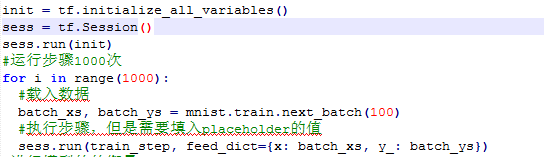8）模型评估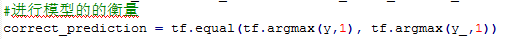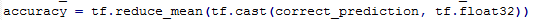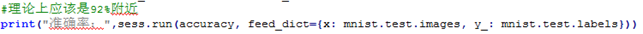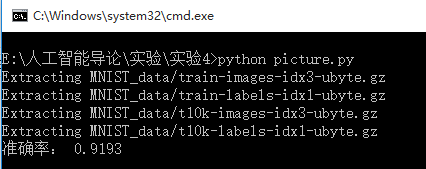【卷积神经网络CNN实现图像识别】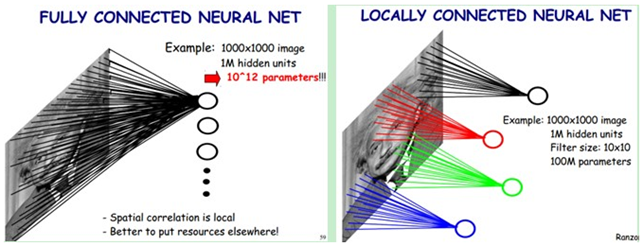卷积神经网络与普通神经网络的区别在于，卷积神经网络包含了一个由卷积层和子采样层构成的特征抽取器。在卷积神经网络的卷积层中，一个神经元只与部分邻层神经元连接。在CNN的一个卷积层中，通常包含若干个特征平面(featureMap)，每个特征平面由一些矩形排列的的神经元组成，同一特征平面的神经元共享权值，这里共享的权值就是卷积核。卷积核一般以随机小数矩阵的形式初始化，在网络的训练过程中卷积核将学习得到合理的权值。共享权值（卷积核）带来的直接好处是减少网络各层之间的连接，同时又降低了过拟合的风险。子采样也叫做池化（pooling），通常有均值子采样（mean pooling）和最大值子采样（max pooling）两种形式。子采样可以看作一种特殊的卷积过程。卷积和子采样大大简化了模型复杂度，减少了模型的参数。

卷积神经网络由三部分构成。第一部分是输入层。第二部分由n个卷积层和池化层的组合组成。第三部分由一个全连结的多层感知机分类器构成。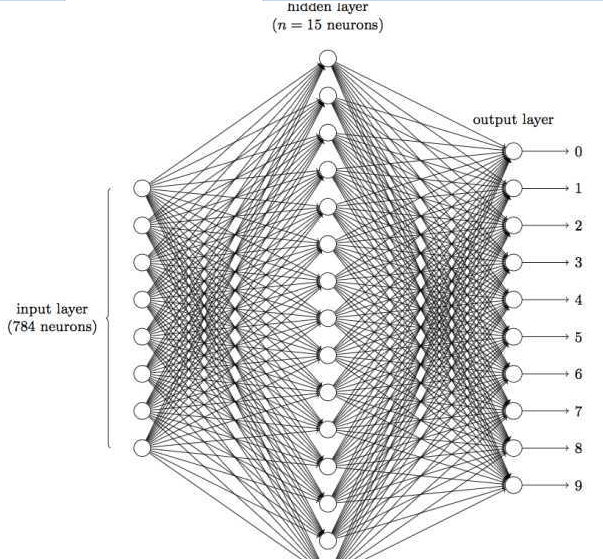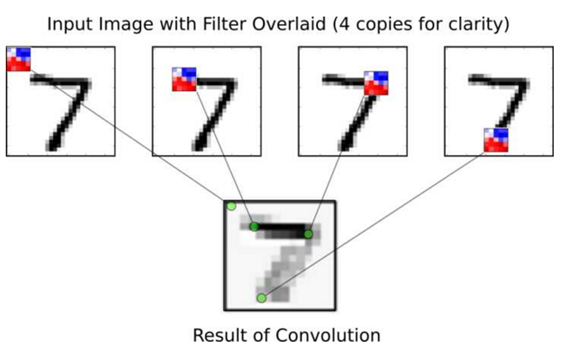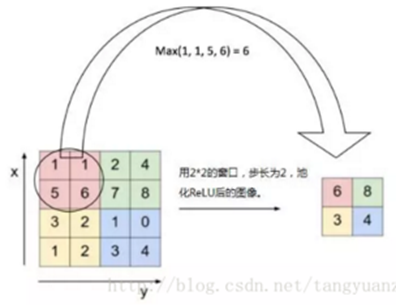Dropout层

dropout是一种防止模型过拟合的技术，这项技术也很简单，但是很实用。它的基本思想是在训练的时候随机的dropout(丢弃)一些神经元的激活，它不会太依赖某些局部的特征（因为局部特征有可能被丢弃）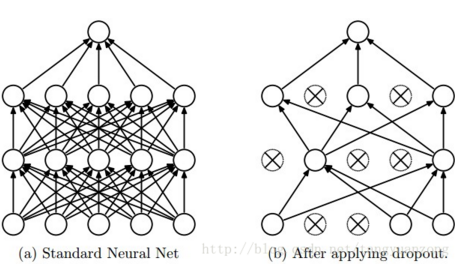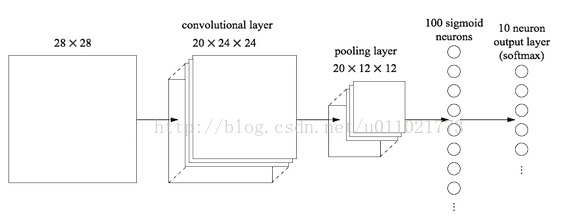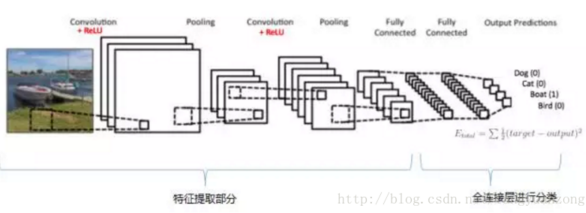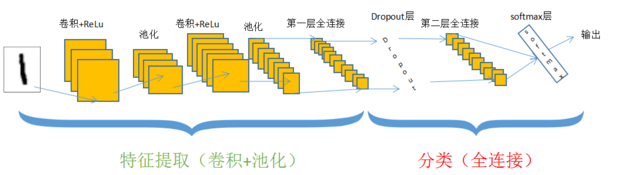【具体实现】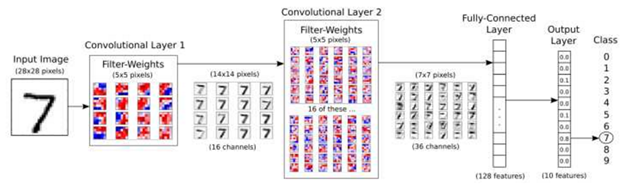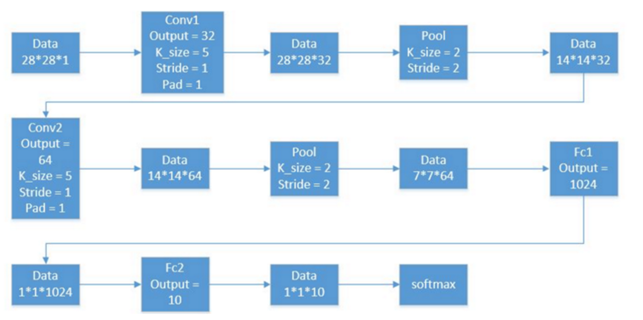1）导入tensorflow包

2）下载数据

3）创建会话

4）为输入的样本特征x与标签y建立占位符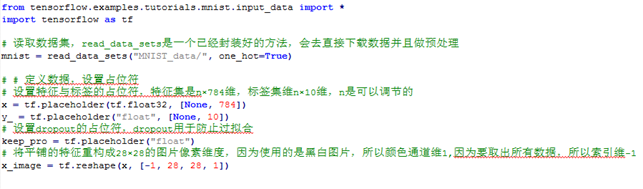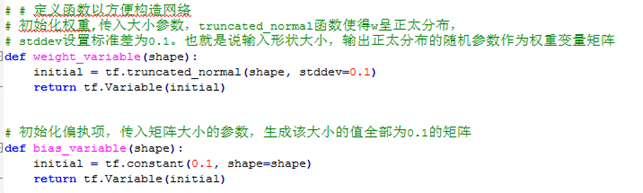Tensorflow也在卷积层与池化层给了很多灵活的操作。卷积层与池化层都需要设置一些超参数，比如步长，窗口大小，补全的边界等。在这里选择使用步长为1，用0补全边界，故卷积层的输出与输入的数据大小是一致的。在池化层中，使用2*2的窗口选取窗口中的最大值作为输出。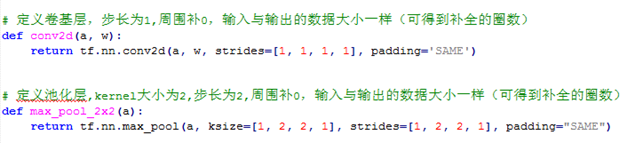第一组卷积层与池化层 这层卷积层总共设置32个神经元，也就是有32个卷积核去分别关注32个特征。窗口的大小是5×5,所以指向每个卷积层的权重也是5×5,因为图片是黑白色的，只有一个颜色通道，所以总共只有1个面，故每个卷积核都对应一组5*5 * 1的权重。因此w权重的tensor大小应是[5,5,1,32] ，b权重的tensor大小应是[ 32 ] ，初始化这两个权重：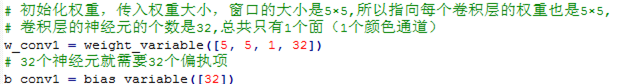在CNN中，我们需要将其组合成28 * 28，因为只有一个颜色通道，确切的来说应该是28 * 28 * 1的数组，-1表示取出所有的数据。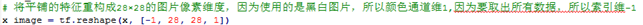将权重w与样本特征x放进卷积层函数中做卷积运算，然后加上偏置项b。将线性输出进入relu激活函数，激活函数的输出就是卷积层的最终输出，输出tensor的大小仍然是28*28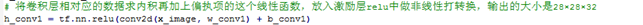卷积层的输出就是接下去池化层的输入,池化层的窗口大小为2*2，故输入的28*28，就变成了输出的14*14大小。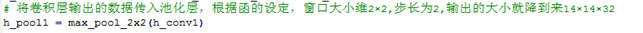第二组卷积层与池化层（类似于第一组） 第二层卷积层中，窗口大小仍然是5 * 5， 神经元的个数是64，因为上一层的神经元个数是32，所以这一次的参数w的大小是[5,5,32,64]，参数b的大小是[ 64 ]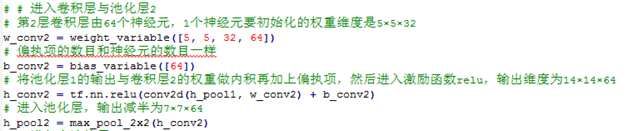经过卷积层tensor的大小是14 * 14,经过池化层tensor的大小变成了7 * 7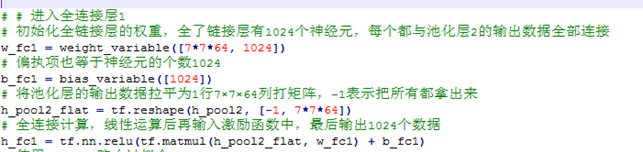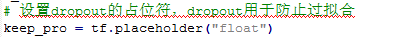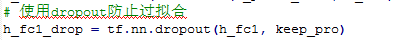keep_prob表示保留不关闭的神经元的比例。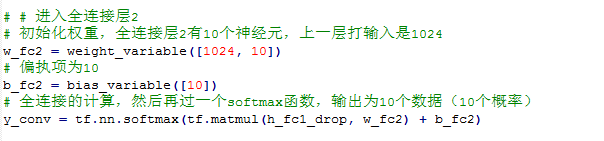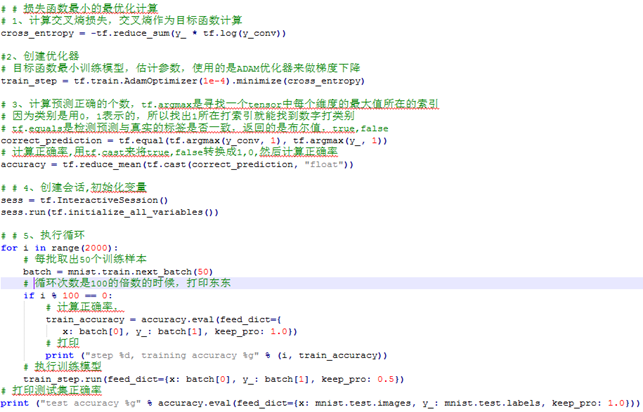2000次循环，每循环100次，会打印出训练集的误差，打印结果如下：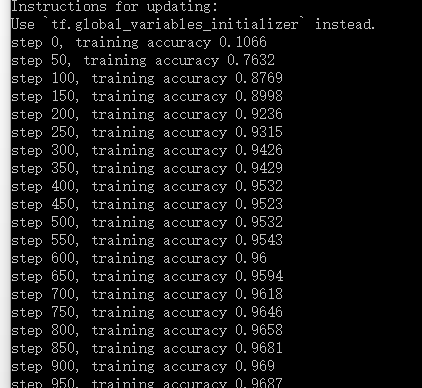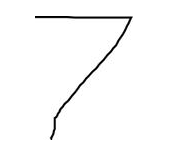【载入图片测试具体步骤】

（1）载入我的手写数字的图像。

（2）将图像转换为黑白（模式“L”）

（3）确定原始图像的尺寸是最大的

（4）调整图像的大小，使得最大尺寸（醚的高度及宽度）为20像素，并且以相同的比例最小化尺寸刻度。

（5）锐化图像。这会极大地强化结果。

（6）把图像粘贴在28×28像素的白色画布上。在最大的尺寸上从顶部或侧面居中图像4个像素。最大尺寸始终是20个像素和4 + 20 + 4 = 28，最小尺寸被定位在28和缩放的图像的新的大小之间差的一半。

（7）获取新的图像（画布+居中的图像）的像素值。

（8）归一化像素值到0和1之间的一个值（这也在TensorFlow MNIST教程中完成）。其中0是白色的，1是纯黑色。从步骤7得到的像素值是与之相反的，其中255是白色的，0黑色，所以数值必须反转。下述公式包括反转和规格化（255-X）* 1.0 / 255.0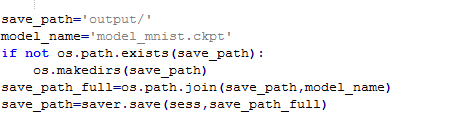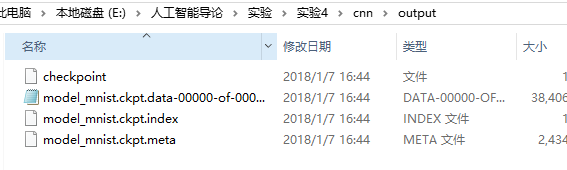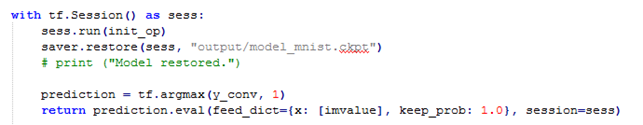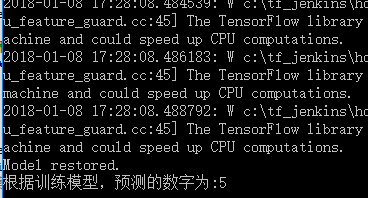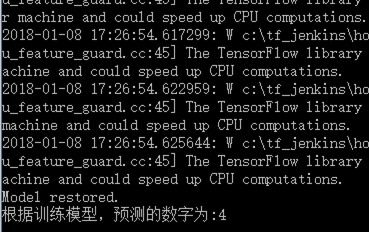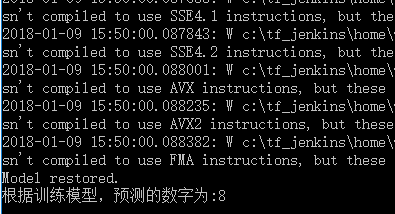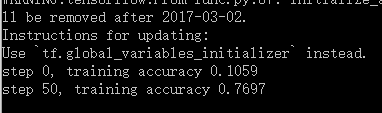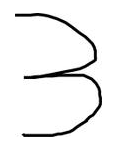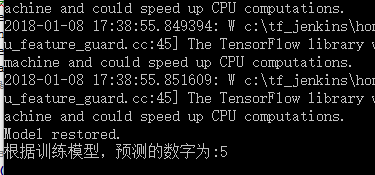Learning Rate（学习率）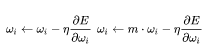Weight decay（权值衰减率）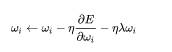weightdecay（权值衰减）的使用既不是为了提高收敛精确度也不是为了提高收敛速度，其最终目的是防止过拟合。在损失函数中，weight decay是放在正则项（regularization）前面的一个系数，正则项一般指示模型的复杂度，所以weight decay的作用是调节模型复杂度对损失函数的影响，若weight decay很大，则复杂的模型损失函数的值也就大。

Momentum （激励值）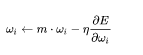momentum是梯度下降法中一种常用的加速技术。对于一般的SGD，其表达式为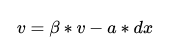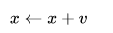normalization（batch normalization）。batch normalization的是指在神经网络中激活函数的前面，将按照特征进行normalization，这样做的好处有三点：

1、提高梯度在网络中的流动。Normalization能够使特征全部缩放到[0,1]，这样在反向传播时候的梯度都是在1左右，避免了梯度消失现象。

2、提升学习速率。归一化后的数据能够快速的达到收敛。

3、减少模型训练对初始化的依赖

Learning Rate Decay （学习衰减率）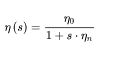重点是卷积神经网络，在对其中的卷积层、池化层、全连接层以及输出层进行深入了解之后，回过头再次来看CNN到底和普通神经网络有什么区别？我觉得可能在于，卷积神经网络中多了个特征抽取步骤。在卷积网络的卷积层中，一个神经元只负责处理图像信息中的特定一部分，就像识别一张人脸，我们可以使用不同的神经元来分别识别眼睛、鼻子、嘴巴或者耳朵等等，所以，在CNN的一个卷积层中，通常包含很多个特征平面，这样的每个特征平面由一些神经元组成，同一个特征平面的神经元共享权值，这里面的权值也就是卷积核。所以卷积层带来的直接好处就是减少了模型的参数。

卷积层进行之后，通常会跟着一个池化层，这里主要有最大池化和均值池化两种，本次实验中使用的是最大池化，在池化过程中，通过特定大小的窗口简化了模型的复杂度，而且同时也保留了对应原图像部分的特征。在具体实现的过程中，我们可以设计多组卷积池化层，这样在对数据集的训练时就可以尽可能地提高正确率，在预测时最大程度上接近真实值。

了解完这次的卷积神经网络之后，这门课的实验就结束了。总的来说，收获还蛮大的，从第一次的几种搜索算法、第二次的决策树等分类算法、第三次的强化学习算法到最后一次的深度学习算法，每一次的实验都是一次对理论知识加深理解、对新知识（weka、python等等）初步认识的过程。确实，仅仅通过课本上的内容，我们很难深入地去体会一些算法到底是怎么具体实现，通过什么来实现的，所以在做完实验之后，会明显地发现自己了解到了更多的知识，虽然过程中会遇到种种问题，或者是搞不懂算法，或者是软件出错，或者是其他各种各样的bug，但最后通过上网查各种资料、通过请教同学等等，还是用心地坚持做下来了。所以，我很感谢这门课的实验让我真正接触到人工智能的美妙之处，同时也激起了我对AI的好奇心，我会接着深入了解下去，带着兴趣。

2016-08-11 14:45:50 Yt7589 阅读数 3717
• ###### 玩转深度学习实战教程

玩转深度学习视频培训课程，详细讲解深度学习的原理和利用深度学习框架TensorFlow进行项目实战。课程通过Kaggle竞赛平台的Titanic问题讲解TensorFlow的基本用法以及问题处理的常用技巧，讲解深度学习图像领域的卷积神经网络CNN和多个经典的网络架构、CNN的应用，讲解自然语言处理领域的RNN、LSTM以及它们的多种变种结构，构建语言模型和对话机器人，介绍损失函数和优化深度学习算法在TensorFlow中的实现。

3209 人正在学习 去看看 王而川

```from __future__ import print_function

__docformat__ = 'restructedtext en'

import six.moves.cPickle as pickle
import gzip
import os
import sys
import timeit

import numpy

import theano
import theano.tensor as T

samplesNumber = 6
features = 2
train_set = (numpy.ndarray(shape=(samplesNumber, features), dtype=numpy.float32), numpy.ndarray(shape=(samplesNumber), dtype=int))
self.prepare_dataset(train_set)
valid_set = (train_set.copy(), train_set.copy())
test_set = (train_set.copy(), train_set.copy())
test_set_x, test_set_y = self.shared_dataset(test_set)
valid_set_x, valid_set_y = self.shared_dataset(valid_set)
train_set_x, train_set_y = self.shared_dataset(train_set)
rval = [(train_set_x, train_set_y), (valid_set_x, valid_set_y),
(test_set_x, test_set_y)]
return rval

def shared_dataset(self, data_xy, borrow=True):
data_x, data_y = data_xy
shared_x = theano.shared(numpy.asarray(data_x,
dtype=theano.config.floatX),
borrow=borrow)
shared_y = theano.shared(numpy.asarray(data_y,
dtype=theano.config.floatX),
borrow=borrow)
return shared_x, T.cast(shared_y, 'int32')

def prepare_dataset(self, dataset):
dataset = 1.0
dataset = 1.0
dataset = 1

dataset = 2.0
dataset = 2.0
dataset = 1

dataset = 3.0
dataset = 3.0
dataset = 1

dataset = 1.5
dataset = 2.0
dataset = 0

dataset = 2.5
dataset = 4.0
dataset = 0

dataset = 3.5
dataset = 7.0
dataset = 0```

```from __future__ import print_function

__docformat__ = 'restructedtext en'

import six.moves.cPickle as pickle
import gzip
import os
import sys
import timeit

import numpy

import theano
import theano.tensor as T
from logistic_regression import LogisticRegression

class LrSegEngine(object):
def __init__(self):
print("Logistic Regression MNIST Engine")
self.learning_rate = 0.13
self.n_epochs = 1000
self.batch_size = 1 # 600
self.dataset = 'mnist.pkl.gz'

def train(self):
print("Yantao:train the model")
train_set_x, train_set_y = datasets
valid_set_x, valid_set_y = datasets
test_set_x, test_set_y = datasets
n_train_batches = train_set_x.get_value(borrow=True).shape // self.batch_size
n_valid_batches = valid_set_x.get_value(borrow=True).shape // self.batch_size
n_test_batches = test_set_x.get_value(borrow=True).shape // self.batch_size
index = T.lscalar()
x = T.matrix('x')
y = T.ivector('y')
# in:x,y out: 1 in y=x otherwise 0
classifier = LogisticRegression(input=x, n_in=2, n_out=2)
cost = classifier.negative_log_likelihood(y)
test_model = theano.function(
inputs=[index],
outputs=classifier.errors(y),
givens={
x: test_set_x[index * self.batch_size: (index + 1) * self.batch_size],
y: test_set_y[index * self.batch_size: (index + 1) * self.batch_size]
}
)
validate_model = theano.function(
inputs=[index],
outputs=classifier.errors(y),
givens={
x: valid_set_x[index * self.batch_size: (index + 1) * self.batch_size],
y: valid_set_y[index * self.batch_size: (index + 1) * self.batch_size]
}
)
updates = [(classifier.W, classifier.W - self.learning_rate * g_W),
(classifier.b, classifier.b - self.learning_rate * g_b)]
train_model = theano.function(
inputs=[index],
outputs=cost,
givens={
x: train_set_x[index * self.batch_size: (index + 1) * self.batch_size],
y: train_set_y[index * self.batch_size: (index + 1) * self.batch_size]
}
)
patience = 5000
patience_increase = 2
improvement_threshold = 0.995
validation_frequency = min(n_train_batches, patience // 2)
best_validation_loss = numpy.inf
test_score = 0.
start_time = timeit.default_timer()
done_looping = False
epoch = 0
while (epoch < self.n_epochs) and (not done_looping):
epoch = epoch + 1
for minibatch_index in range(n_train_batches):
minibatch_avg_cost = train_model(minibatch_index)
# iteration number
iter = (epoch - 1) * n_train_batches + minibatch_index
if (iter + 1) % validation_frequency == 0:
# compute zero-one loss on validation set
validation_losses = [validate_model(i)
for i in range(n_valid_batches)]
this_validation_loss = numpy.mean(validation_losses)
print(
'epoch %i, minibatch %i/%i, validation error %f %%' %
(
epoch,
minibatch_index + 1,
n_train_batches,
this_validation_loss * 100.
)
)
if this_validation_loss < best_validation_loss:
#improve patience if loss improvement is good enough
if this_validation_loss < best_validation_loss * improvement_threshold:
patience = max(patience, iter * patience_increase)
best_validation_loss = this_validation_loss
# test it on the test set
test_losses = [test_model(i)
for i in range(n_test_batches)]
test_score = numpy.mean(test_losses)
print(
(
'     epoch %i, minibatch %i/%i, test error of'
' best model %f %%'
) %
(
epoch,
minibatch_index + 1,
n_train_batches,
test_score * 100.
)
)
# save the best model
with open('best_model.pkl', 'wb') as f:
pickle.dump(classifier, f)
if patience <= iter:
done_looping = True
break
end_time = timeit.default_timer()
print(
(
'Optimization complete with best validation score of %f %%,'
'with test performance %f %%'
)
% (best_validation_loss * 100., test_score * 100.)
)
print('The code run for %d epochs, with %f epochs/sec' % (
epoch, 1. * epoch / (end_time - start_time)))
print(('The code for file ' +
os.path.split(__file__) +
' ran for %.1fs' % ((end_time - start_time))), file=sys.stderr)

def run(self, data):
print("run the model")
predict_model = theano.function(
inputs=[classifier.input],
outputs=classifier.y_pred
)
rst = predict_model(data)
print(rst)
```

```from __future__ import print_function

__docformat__ = 'restructedtext en'

import six.moves.cPickle as pickle
import gzip
import os
import sys
import timeit

import numpy

import theano
import theano.tensor as T

class LogisticRegression(object):
def __init__(self, input, n_in, n_out):
self.W = theano.shared(
value=numpy.zeros(
(n_in, n_out),
dtype=theano.config.floatX
),
name='W',
borrow=True
)
self.b = theano.shared(
value=numpy.zeros(
(n_out,),
dtype=theano.config.floatX
),
name='b',
borrow=True
)
self.p_y_given_x = T.nnet.softmax(T.dot(input, self.W) + self.b)
self.y_pred = T.argmax(self.p_y_given_x, axis=1)
self.params = [self.W, self.b]
self.input = input
print("Yantao: ***********************************")

def negative_log_likelihood(self, y):
return -T.mean(T.log(self.p_y_given_x)[T.arange(y.shape), y])

def errors(self, y):
if y.ndim != self.y_pred.ndim:
raise TypeError(
'y should have the same shape as self.y_pred',
('y', y.type, 'y_pred', self.y_pred.type)
)
if y.dtype.startswith('int'):
return T.mean(T.neq(self.y_pred, y))
else:
raise NotImplementedError()
```

```from __future__ import print_function

__docformat__ = 'restructedtext en'

import six.moves.cPickle as pickle
import gzip
import os
import sys
import timeit

import numpy

import theano
import theano.tensor as T

from logistic_regression import LogisticRegression
from lr_seg_engine import LrSegEngine

if __name__ == '__main__':
engine = LrSegEngine()
engine.train()
```

```from seg_loader import SegLoader
from lr_seg_engine import LrSegEngine

if __name__ == '__main__':
print("test program v1.0")
engine = LrSegEngine()
data = [[2.0, 2.0]]
print(data)
engine.run(data)
```

test program v1.0

Logistic Regression MNIST Engine

[[2.0, 2.0]]

run the model